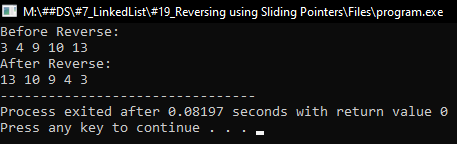## How to Reverse a Linked List by Reversing Links in C Language:

In this article, I am going to discuss How to Reverse a Linked List by Reversing Links in C Language with Examples. Please read our previous article, where we discussed How to Reverse a Linked List in C Language with Examples.

Here, we will reverse a linked list by reversing the links of the nodes.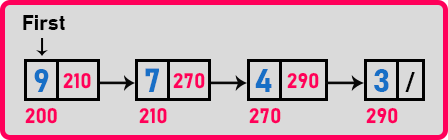In this linked list, node ‘9’ is pointing to node ‘7’, and node ‘7’ is pointing to node ’4’. Here reversing the links means node ‘7’ will be pointing to node ‘9’ instead of pointing to node ‘4’. By reversing the links, we will get the linked list in reverse order as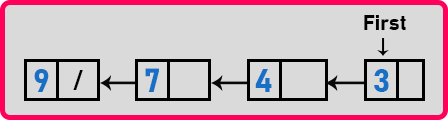Now before showing you how to reverse links of a linked list, I will show you one small concept that is sliding pointers. What is meant by sliding pointers? Let us see. We are taking three-pointers as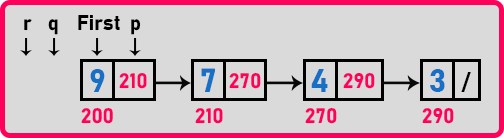The pointer ‘p’ is pointing on the first node, ‘q’ is null and ‘r’ is also null. This is the initial state means we have just initialized the pointers but we want sliding pointers or tailing pointers. In the tailing pointer, one pointer will follow the second pointer. Here are 3 pointers so we are calling them sliding pointers. So let us see how we want them to work.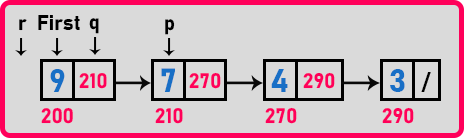Here we have moved ‘r’ to ‘q’ position, ‘q’ to ‘p’ position, and ‘p’ to ‘p->next’. So, we just move all the pointers by one node. Now ‘q’ is pointing on the first node, ‘p’ is pointing on the 2nd node and ‘r’ is null. Moving all the three-pointers is a single step. All three are moving together that’s why we are calling them sliding pointers.

So, in this way we can move 3 pointers together. We want to move all the pointer until ‘p’ becomes null. We can write the code for moving sliding pointer as,

p = first;
q = NULL;
r = NULL;
while(p != NULL){
r = q;
q = p;
p = p->next;
}

So, in this way we can move three points together. Now let us see how we can reverse the links. Let us take a small example and show you what are the requirements for reversing the links. Suppose we have 3 nodes,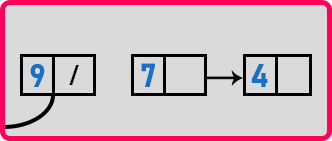Here the first is pointing to the previous node. Now we have to reverse the link of the next node i.e. ‘7’ so that it will point to the previous node i.e. ‘9’. We have to modify this one. So let us take a pointer ‘q’ on node ’7’ as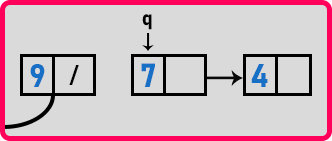Now for changing q’s link we should know the address of the previous node i.e. ‘9’. Let us take another pointer ‘r’ in the previous node i.e. ‘9’ as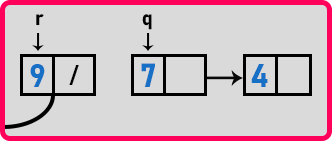When this link of ‘q’ is modified then this will be pointing to ‘r’ as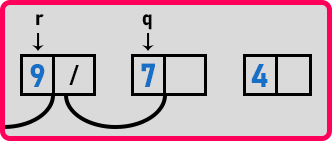And now we haven’t the address of the next node i.e. ‘4’. Here we require another pointer ‘p’ which will point to the next node from the current node.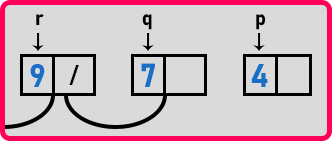We need 3 pointers that are the node that is modified, the node previous to the current node, and the next node from the current node. Now we have to modify the p’s link so for that we have to move ‘q’ to there and also ‘r’ and ‘p’. So, we just move all the pointers by one node as,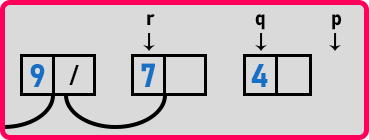It means that ‘p’, ‘q’, and ‘r’ are sliding so that is the reason we are calling them sliding pointers. So, slide and reverse the links. Let us add a single statement to the previous code as

p = first;
q = NULL;
r = NULL;
while(p != NULL){
r = q;
q = p;
p = p->next;
q->next = r;
}

Here we add ‘q->next = r’; Now let us trace these pointers on the above example.

##### Tracing of Pointers:In the starting ‘p’ is pointing on the first node and ‘r’ and ‘q’ are pointing on NULL. Here ‘p’ is not NULL so move all the pointers to next node as,Now modify ‘q->next = r’. ‘r’ is pointing on null so ‘q->next’ will be null.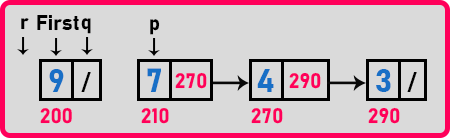This is the first step. Here ‘p’ is not NULL so move all the pointers by one node as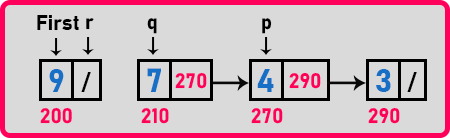Here ‘p’ is pointing on ‘4’, ‘q’ is pointing on ‘7’ and ‘r’ is pointing on ‘9’. Now modify q’s link as ‘q->next = r’ so q will point on r as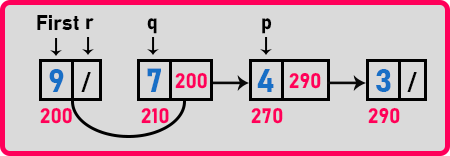Here ‘q->next’ is pointing on ‘r’. In this way, we will change the links to a linked list. After reversing all the links, the linked list will be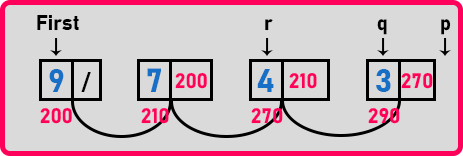Here ‘q’ is the first node so we have to add a statement ‘first = q’ in our code. So, these are the simple steps for reversing a linked list. As we already discussed the movement of data is not preferred in a linked list so we prefer to reverse the links of a linked list. The reason behind this is that for learning purposes we are just taking integers only but it may be integers or maybe not. It can be a huge record. So, at that time we will not change the records. So that’s why it is preferred to reverse links not the data in a linked list. Now let us write complete program for reversing a linked list.

##### Program to Reverse a Linked List by Reversing Links using C Language:
```#include <stdio.h>
#include <stdlib.h>

struct Node
{
int data;
struct Node *next;
} *first = NULL;

void Create(int A[], int n)
{
int i;
struct Node *t, *last;
first = (struct Node *) malloc(sizeof(struct Node));
first->data = A;
first->next = NULL;
last = first;

for (i = 1; i < n; i++)
{
t = (struct Node *) malloc(sizeof(struct Node));
t->data = A[i];
t->next = NULL;
last->next = t;
last = t;
}
}

void Display(struct Node *p)
{
while (p != NULL)
{
printf ("%d ", p->data);
p = p->next;
}
}

{
struct Node *q = NULL, *r = NULL;
while (p != NULL)
{
r = q;
q = p;
p = p->next;
q->next = r;
}
first = q;
}

int main()
{
int A[] = { 3, 4, 9, 10, 13, 16, 19 };
Create (A, 5);

printf("Before Reverse: \n");
Display(first);